# Explain Logic OR Gate and Its operation with Truth Table

## OR Gate

The OR gate performs logic addition, more commonly known as the OR function.

An OR gate has two or more input signals with only one output signal.

In OR gate, output voltage is high if any or all of the input voltages are high.

Diodes may be used to build an OR gate, as shown in the Fig 1 .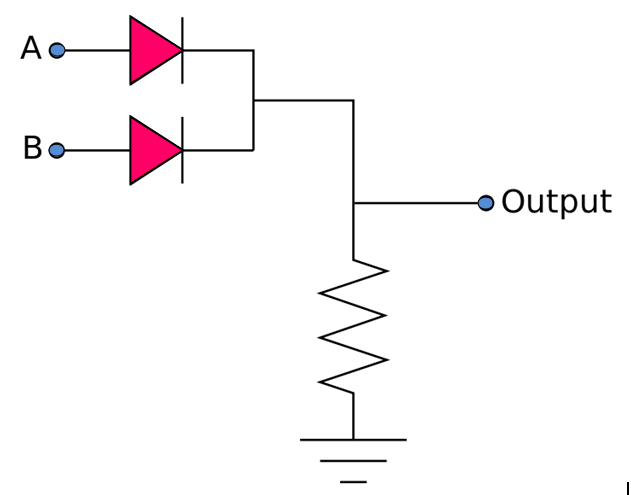Fig 1

If input voltages at points A, B are low then all diode are non-conducting so output voltage, Vout is low . But if any of the input terminal is at high voltage, then diode connected with that particular terminal is forward biased and current flows through the resistance R. The result is that Vout is at high level.

### OR Gate Symbol

The symbols used for OR gates with 2,3 and N inputs are shown in Fig 2 (a), (b) and (c) respectively.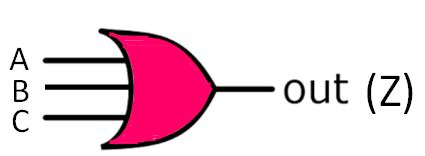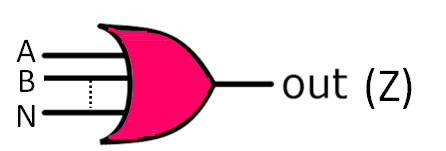Fig.2 (a)                                           Fig.2 (b)                                                         Fig.2 (c)

The inputs A, B, C…N are logic voltage levels and the output Z is a logic voltage level whose values is the result of the OR operation on A, B, C ….and N.

In other words, the OR gate operates in such a way that its output is high (logic 1) if and only if one or more inputs are high. The OR gate output will be low (logic 0) only if all inputs are low or at logic o.

Its logic equation is given as :

Z = A OR B OR C ….OR N

= A + B + C +…………..+N

The above equation is known as Boolean equation or the logical equation of the OR gate.

### OR Gate Truth Table

The logical operation of  two -input and three-input OR gate is described in truth table shown in table 1 and table 2 respectively .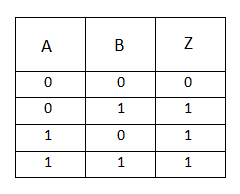Table  1 :Truth table of 2-input OR gate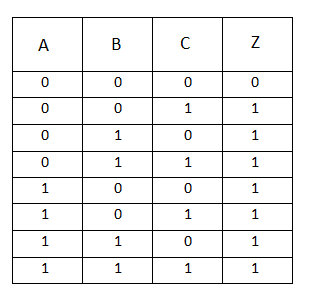Table 2: Truth table of 3-input  OR gate

The truth table can be expanded for any number of inputs; but regardless of the number of inputs, the output is high when any one or more of the inputs are high.

Incidentally, the number of rows in a truth table equals 2n, where n is the number of inputs. For a 2-input OR gate, the truth table has 22 or 4 rows. A 3-input OR gate has 23 i.e. 8 rows and so on.

An OR gate can have as many inputs as desired, add one diode for each additional input. Six diodes result in a 6-input OR gate, twelve diodes in a 12-input OR gate. No matter how many inputs, the action of the OR gate, is summarized like this; one or more high inputs produce a high output.

The OR gate can be thought of as an electrical circuit in which switches are connected in parallel with each other. Lamp will glow [output will be present] when either or all switches are closed.

### Transistor OR Gate

A simple 2-input logic OR gate can be constructed using RTL Resistor-transistor switches connected together as shown below .

Here the inputs  A and B are connected directly to the transistor bases. Either transistor must be saturated “ON” for an output at Z .

Fig. 3 depicts a possible transistor OR gate consisting of two interconnected transistors Tand T2  supplied from a common supply VCC = +5 V.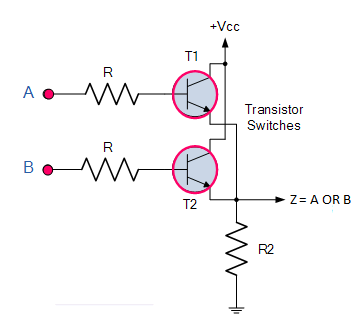Fig. 3

### Standard Package

Figure 4 depicts the pinout diagram of IC7432, a TTL quad 2-input OR gate.Fig. 4

It has a 14-pin dual-inline package (DIP) and has four 2-input OR gates. After connecting a supply voltage of +5 V to pin 14 and a ground to pin 7, we may connect one or more of the OR gates to other TTL devices.

### Pulsed Operation

In many applications, the inputs of OR gate may be in the form of pulses ( not in the form of single bit).

The output depends upon the relationship between different pulses.

Figure 5 illustrates two pulses A and B as inputs to an OR gate.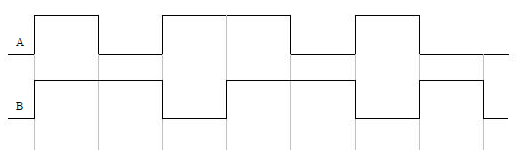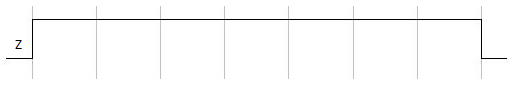Fig. 5

The output Z  of the OR gateis also shown in the above figure.

In the given waveform during the first time interval both inputs are high so output Z is high.

During the second time interval  input A is low but B is high, so output Z is high.

During third time interval  input A is high but B is low,  so output Z is again high .

Similarly, the outputs for the remaining time intervals are calculated and the waveform  drawn as shown in above figure.